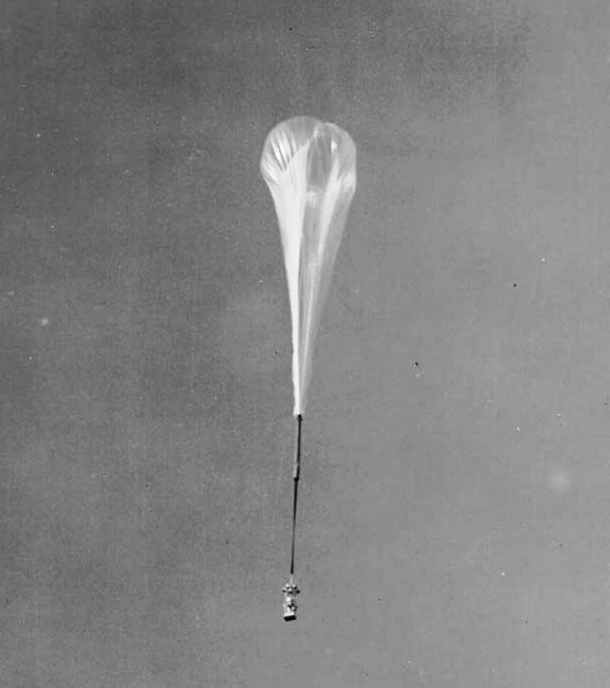# SECRET OF CSS

## NEWS# Python Shorts — Pendulums. Pendulums are nature’s clocks, and a… | by John Clark Craig | Aug, 2022

## Pendulums are nature’s clocks, and a short Python program makes it fun to calculate some fascinating things with them.Skyhook balloon — Courtesy United States Navy

I’ve seen some interesting things by staring out my big window into the dark blue daytime sky above the Denver skyline. Most balloons have been of the small party type, usually accidentally released by a careless youngster somewhere. But one afternoon a high-altitude upside-down tear-drop-shaped balloon appeared, very similar in appearance to the Skyhook balloons of decades past, as shown above.

I couldn’t tell how big the balloon was, but I could tell it was up at a very high altitude. Its barely visible payload was hanging quite a way below and it was swinging back and forth slowly.

It suddenly dawned on me that I could measure the balloon in a very unique way, even from that great distance. So I did. My rough but reasonable calculation showed the payload was hanging something like 70 feet below the balloon at the end of a long tether.

Fast forward to yesterday. I was sitting in a coffee shop situated in an old antique firehall building near downtown Denver. Several chandelier type lamps were hanging from the very high ceiling, and I noticed one was swinging back and forth ever so slightly. I measured the length of the lamp using the same technique as I had years ago with the balloon, and it worked out to about 12 feet. (Yes, I was bored, but this did help the time go by gracefully!)

Both of those calculations used a simple formula for the swing time of a pendulum, of course. It turns out that it doesn’t matter how heavy a mass at the end of a string or cord is. The period of its swing depends only on the length of that string or cord. If you know its length, you can calculate a pendulum’s period, or the time it takes to swing back and forth once, or if you know its period you can calculate its length.

Here’s the formula that summarizes these calculations, where T is the time for one full swing, or period, of a pendulum, and L is the length of the string or cord. Notice that g is the acceleration of gravity, which is about 9.8 meters per second per second on earth.

Here’s my short Python program that lets you input either the period of swing, or the length of swing, and the other will be calculated.

`import mathprint("\nPENDULUM")print("period in seconds")print("length in meters")print("\nEnter one value..\n")seconds = input(s := "Period: ")seconds = float(seconds) if seconds else 0.0meters = input(m := "Length: ")meters = float(meters) if meters else 0.0if seconds:meters = 9.8 * (seconds / math.pi / 2) ** 2else:seconds = 2 * math.pi * (meters / 9.8) ** 0.5print()print(s, seconds, "seconds")print(m, meters, "meters")print(m, meters * 100 / 30.48, "feet")print(m, meters * 100 / 2.54, "inches")`

## Python Talking Points

Take a look at the two lines of code where the user enters seconds. The walrus operator `:=` is used to set the variable named `s` to `"Period: "` so this string can be reused in the output lines near the end.

The next code line sets the value of seconds to the float value of whatever the user types, or to zero if nothing was typed in. This is a handy little input trick I use for a lot of simple programs.

If seconds are input then the meters length for the pendulum in question is calculated. Otherwise, if the meter’s length is input then the number of seconds for the pendulum’s period is calculated.

Notice that the two lines of calculations use the double asterisk `**` for finding both the square and the square roots of values. Raising a number to the power of 0.5 is the same as finding the square root. Doing it this way does not require importing the math module, although in this program we need to import math anyway, to access the value of pi.

As a working example, imagine you have some fishing string, a pocketknife, and a 3×5 note card, and you are out in the woods somewhere at the base of a very tall cliff you want to measure.

After playing with this program at home, before you left for the woods, you recall that a pendulum of 39 inches has a period of 2 seconds. Here’s what that sample calculation looks like using the program above…

Okay, here’s what you do to measure the height of the cliff.

While your friend climbs to the top of the cliff, you tie the string to the pocketknife and measure the string to 39 inches (7 lengths of the card, 1 width, and another 1/3 of its width).

Your friend drops a rock and you time the number of seconds it takes to hit the ground. Presto magico, you have all you need to calculate the height of the cliff! (I’ll leave the rest of that calculation for you to discover.)

## Word of Caution

Be sure to watch your friend, the pendulum, and the rock with care. Enough said.